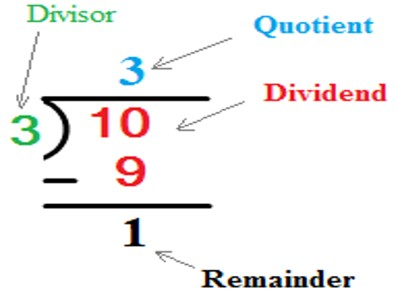Division Terms

There are four terms which describe the four numbers in a division problem. The dividend is the number that is being divided. The divisor is the number that the dividend will be divided by. The quotient is the number of times the divisor will go into the dividend. The remainder is a number that is less than the divisor and is too small to be divided by the divisor to form a whole number.

In division, a dividend is divided by a divisor to find a quotient.

In the following equation, 18 is the dividend, 3 is the divisor, and 6 is the quotient.

18 / 3 = 6

If there is an amount left over, it is called the remainder. The remainder cannot be evenly divided by the divisor. For example, if you divide 18 by 7, you will get a remainder:

18 / 7 = 2, with a remainder of 4.Explanation

A division problem may be written horizontally such as 49474 / 7 = 7067 R 5. In this example 7 is the divisor, 49474 is the dividend, 7067 is the quotient and 5 is the remainder of the division.

A division problem may be written vertically.

Again, 7 is the divisor, 49474 is the dividend, 7067 is the quotient and 5 is the remainder of the division.

When beginning the long division algorithm, realize that you are asking questions such as “what number times 6 is less than or equal to 300?” Remember that the 3 is in the hundreds place. Since the answer is 5 tens, or 50, a 5 can be written above the 0 in the tens place as shown below. Since 5 tens = 50, and 50 times6 = 300, we can take 300 away from 306 leaving 6. The process is repeated when asking “what number times 6 is less than or equal to 6.” Since the answer is 1, a 1 is written above the 6 in the ones place. Since 6 times1 is 6, 6 is taken away from 6 leaving 0. The problem is complete since the amount left over, the remainder, is less than the divisor 6.

Each of the terms used in division are explained below:

• The number which is divided is called the dividend.
• The number which divides is called the divisor.
• The number which is the result of the division is called the quotient.
• If there is any number left over, it is called the remainder.

Information Source: